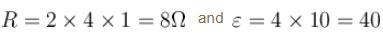Courses

# Electromagnetic Induction NAT Level - 2

## 10 Questions MCQ Test Topic wise Tests for IIT JAM Physics | Electromagnetic Induction NAT Level - 2

Description
This mock test of Electromagnetic Induction NAT Level - 2 for IIT JAM helps you for every IIT JAM entrance exam. This contains 10 Multiple Choice Questions for IIT JAM Electromagnetic Induction NAT Level - 2 (mcq) to study with solutions a complete question bank. The solved questions answers in this Electromagnetic Induction NAT Level - 2 quiz give you a good mix of easy questions and tough questions. IIT JAM students definitely take this Electromagnetic Induction NAT Level - 2 exercise for a better result in the exam. You can find other Electromagnetic Induction NAT Level - 2 extra questions, long questions & short questions for IIT JAM on EduRev as well by searching above.
*Answer can only contain numeric values
QUESTION: 1

### A wire of fixed length is wound on a solenoid of length ‘l ’ and radius ‘r ’. Its self inductance is found to be L. Now if same wire is wound on a solenoid of length l/2 and radius r/2 then the self inductance will be  nL. Find the value of n.

Solution: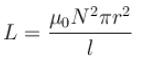Length of wire =  N2πr = Constant (= C, suppose)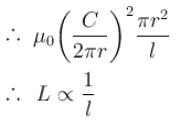∴   Self inductance will become  2L.

*Answer can only contain numeric values
QUESTION: 2

### The number of turns, cross-sectional area and length for four solenoids are given in the following table.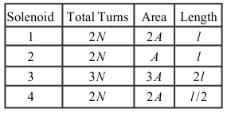The solenoid with maximum self inductance is :

Solution: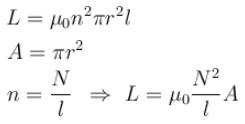By putting the given values, it can be seen that it is maximum for solenoid number 4.

*Answer can only contain numeric values
QUESTION: 3

### In an ideal transformer, the voltage and the current in the primary are 200 volt and 2amp respectively. If the voltage in the secondary is 2000 volt. Then value of current (in Amp) in the secondary will be :

Solution:

Voltage in primary     vp = 200volt

Current in primary     ip  = 2amp

Voltage in secondary  vs = 200volt

The relation for the current in the secondary is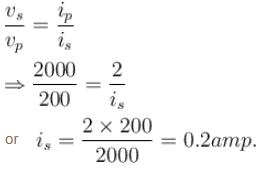*Answer can only contain numeric values
QUESTION: 4

In a L-R  growth circuit, inductance and resistance used are 1H and 20Ω respectively. If at  t = 50 millisecond, current in the circuit is 3.165A then applied direct current emf (in volt) is :

Solution: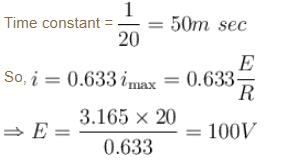*Answer can only contain numeric values
QUESTION: 5

A long coaxial cable consists of two thin walled conducting cylinders with inner radius 2cm and outer radius 8cm. The inner cylinder carries a steady current 1A, and the outer cylinder provides the return path for that current. The current produces a magnetic field between the two cylinders. Find the energy stored in the magnetic field for length 1m of the cable. Express answer in nJ (use ln2 = 0.7)

Solution:

The magnetic field inside is only due to current of inner cylinder.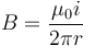Magnetic field energy density is not uniform in the space in between cylinders. At distance r from the centre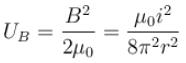Energy in volume of element (length l)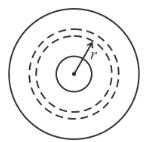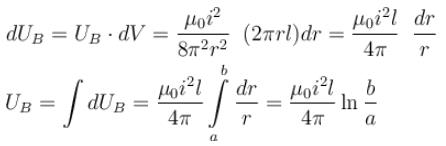Using values U = 140 nJ

*Answer can only contain numeric values
QUESTION: 6

A uniform magnetic field exists in region given by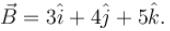A rod of length 5m is placed along y-axis is moved along x-axis with constant speed  1m/sec. Then induced emf (in volts) in the rod will be :

Solution: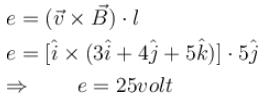*Answer can only contain numeric values
QUESTION: 7

Figure shows a square loop of side 1m and resistance . The magnetic field on left side of line PQ has a magnitude  B = 1.0T. The work done (in Joule)  in pulling the loop out of the field uniformly in 1s  is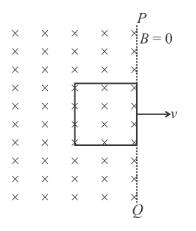Solution: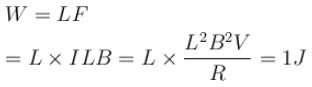*Answer can only contain numeric values
QUESTION: 8

The magnetic field of a cylindrical magnet that has a pole-face radius 2.8cm  can be varied sinusoidally between minimum value 16.8T  and maximum value 17.2T  at a frequency of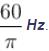Cross section of the magnetic field created by the magnet is shown. At a radial distance of 2cm  from the axis find the amplitude of the electric field (in  mN/C) induced by the magnetic field variation.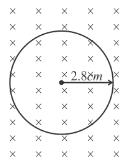Solution: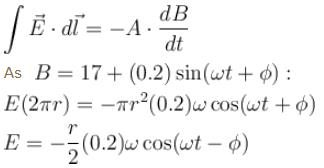Magnitude of the amplitude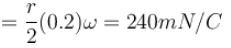*Answer can only contain numeric values
QUESTION: 9

In the figure, a long thin wire carrying a varying current lies at a distance y above one edge of a rectangular wire loop of length L and width W  lying in the x-z plane. If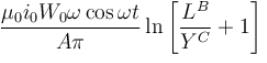emf is induced in the loop. Find the value of (A+B+C)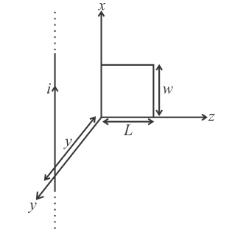Solution:The magnetic field at point  P, is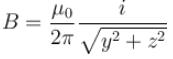The magnetic flux through the shaded strip, is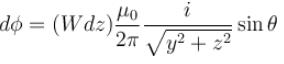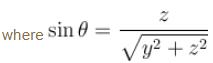∴  total magnetic flux through rectangular loop is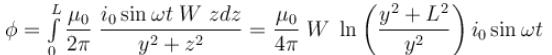∴  induced emf in the loop is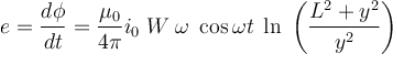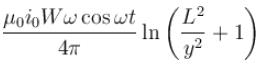*Answer can only contain numeric values
QUESTION: 10

In the figure shown ABCDEFGH  is a square conducting frame of side 2m and  resistance 1Ω/m·A uniform magnetic field B  is applied perpendicular to the plane and pointing inwards. It increases with time at a constant rate of  10T/s. Find the rate at which heat in watt is produced in the circuit  AB = BC = CD = BH.

Solution: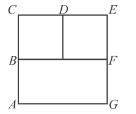The induced emf in loop ABHFG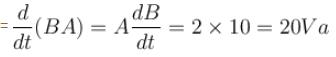The induced emf in loop BCDH  and DEFH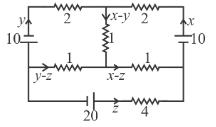= 1 × 10 = 10 volt   KVL  in top left loop is
= 1 × 10 = 10 volt
10 – (y – z) + (x – y) - 2y = 0

⇒  x – 4y + z = – 10    ...(1)

KVL in right loop : 10 – 2x – (x – y) – (x – z) = 0

⇒  –4x + y + z = –10    ...(2)

By equation (1) and (2) it is seen that  x – y = 0  ⇒   no current in DH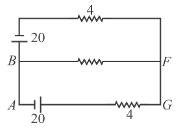(This can also be seen by symmetry)

This makes solution very simple now the circuit is,
Assume  VB = 0, and  VF = V,  then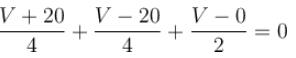⇒  V = 0   ⇒  no current in FB

∴  circuit is :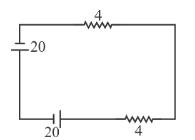∴   rate of heat production = (40)2/8 = 200 Watt.

Note : If you are not able to observe the symmetry or decide
x – y = 0, then write KVL  in the lower loop. It will be  x + y – 6z = –20    ...(3)
Solving (1), (2) and (3) you will get x = +5, y = 5, = 5A. Heat rate will be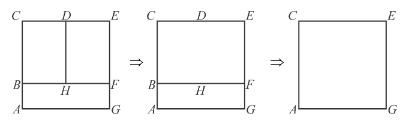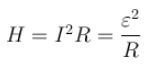where